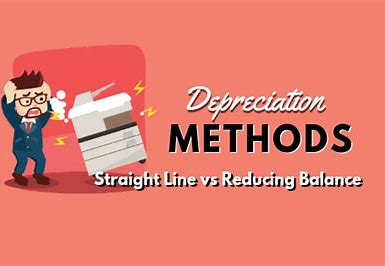# Depreciation of Fixed Assets II

Hello!

Welcome to today’s class. We will be learning about the various methods of DepreciationBy the end of this lesson, you should be able to;

• Graphically represent the methods of depreciation
• Describe in details: Straight Line method and Reducing the Balance method
• Compare and contrast the merits and demerits of each method

NOTE

##### METHODS OF PROVIDING FOR DEPRECIATION
1. Straight-line Method
2. Reducing Balance Method (or Diminishing Balance Method)
3. Sum of the year’s digit
4. Revaluation Method
5. Depletion Unit Method

Other less common methods include:

1. Sinking Fund Method
2. Insurance Policy Method
3. Annuity Method etc.
##### STRAIGHT LINE METHOD

Under this method, an equal amount is charged for depreciation yearly throughout the usage of an asset.

Formula =     Cost – Scrap Value

No. of years

Illustration:

The cost of a machine is N50,000. The residual value is N8,000 and is expected to last for 7 years. Calculate the depreciation charge for each of the seven-year.

Solution

Depreciation   =    Cost – Scrap Value

Estimated Useful life

=    50,000 – 8,000

7

=    42,000/7

=  N6,000

N.B.      The N6,000 is debited to Profit and Loss Account and credited to the Provision for Depreciation on Machine Account

###### ADVANTAGES OF THE STRAIGHT LINE METHOD
1. It is simple (or easy) to calculate
2. It is widely used
3. It is time-oriented
4. It is judicially recommended – Edwards v. Sauntons Hotels
###### DISADVANTAGES OF THE STRAIGHT LINE METHOD
1. It is not suitable (or ideal) for all type of fixed assets. For example, it cannot be used for loose tools
2. It is not scientific – as it does not take the efficiency of the asset into consideration
3. The assumption of equal depreciation per year is unrealistic.

#### REDUCING BALANCE METHOD (or DIMINISHING BALANCE METHOD)

Under this method, a fixed percentage is written off the reducing (or diminishing) balance of the asset yearly. This method charges higher depreciation in the early years of the asset and lowers in the later years

The depreciation rate (%) to be applied is computed using the formula below:

S

Depreciation rate (%)   =    1   –     C

where:

n =    No. of  years

s  =   Scrap value

c  =   Cost

Illustration:

A machine costing N10,000 will realize N256 in four years. Show the yearly depreciation to be charged for each of the four years using a diminishing balance method.

SOLUTION:

S

Depreciation Rate (%)    =   1   –       C

256

=   1 –  10,000

4

=   1  –     10

=        0.6

=        60%

The depreciation charge applicable to each of the four years will be:

N

Cost                                                                           10,000

Year 1       Depreciation = (60% X 10,000)                   6,000

REDUCED BALANCE (i.e. NBV)                                     4,000

Year 2      Depreciation = (60% X  4,000)                     2,400

REDUCED BALANCE (i.e. NBV)                                     1,600

Year 3      Depreciation = (60% X 1,600)                         960

REDUCED BALANCE (i.e. NBV)                                        640

Year 4     Depreciation = (60% X 640)                             348

SCRAP VALUE (i.e. NBV)                                                  256

##### ADVANTAGES OF THE DIMINISHING BALANCE METHOD
1. It is widely used.
2. Depreciation is more scientifically provided for.
3. It recognizes the efficiency of an asset by charging higher amounts in the early years and lower amounts in the later years.
4. Higher depreciation amounts and low maintenance cost in the early years even out with low depreciation amounts and higher maintenance cost in the later years
##### DISADVANTAGES OF THE DIMINISHING BALANCE METHOD
1. Calculating the rate of depreciation may be difficult
2. It is not ideal for all fixed assets. For example, it cannot be used for loose tools.

In our next class, we will be talking more about Methods of Depreciation – Sum of the Year Digit and Revaluation method. We hope you enjoyed the class.

Should you have any further question, feel free to ask in the comment section below and trust us to respond as soon as possible.

How Can We Make ClassNotesNG Better - CLICK to Tell Us💃